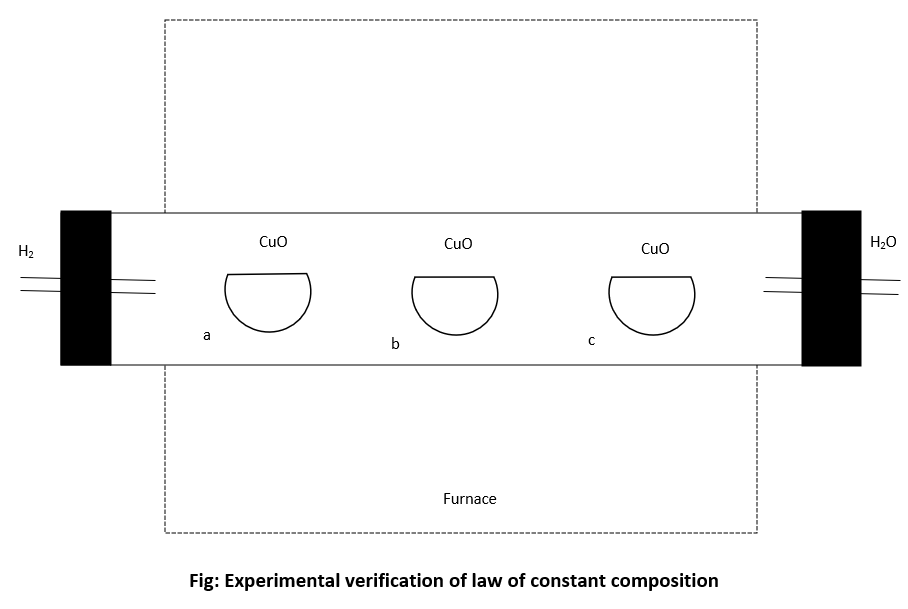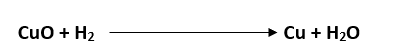# Law of Constant Composition↪ This law is also called the law of definite proportion. This law states," A particular compound always contains the same elements combined to each other in fixed proportion or fixed ratio by mass."

For example: Water always contains hydrogen and oxygen in the ratio of 1:8 by their mass whether it is prepared in lab or obtained from any natural sources.

⁕ ﻿Experimental Verification of Law of Constant CompositionFirst of all known weight of three different samples of CuO which are obtained by different methods are taken in three different porcelain basin and then they are kept inside a tube. The samples are completely reduced into free copper.Then the mass of Cu left in each basin is measured and mass of oxygen present in each oxide sample is determined as:

mass of oxygen = mass of CuO - mass of Cu

If the ratio of mass of Cu and oxygen in all three samples is same then this will verify the law of constant composition or law of definite proportion.